Next: 4.4 Field Distribution of Up: 4 Modelling the Internal Previous: 4.2 Pinning, Thermal Fluctuations,

# 4.3 Gaussian Field Distribution Analysis

Traditionally, the behaviour of the magnetic penetration depth in the vortex state of a superconductor has been determined from the variation of the second moment (i.e. the square of the width) of the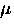SR line shape. The second moment of the local magnetic field distribution n(B) is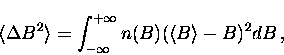(1)
whereis the first moment of n(B) (i.e. the average local magnetic field). At moderate magnetic fields, the second moment of the field distribution for a vortex lattice (which is considered in the next section) has been shown in the London picture to be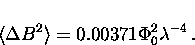(2)
A simple way to model the second moment is to assume that n(B) is a Gaussian distribution of static internal magnetic fields. The corresponding muon polarization function is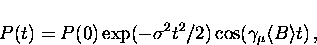(3)
where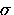is the muon depolarization rate. For a Gaussian field distribution, the second moment is given by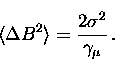(4)
so that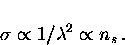(5)
However, this method is extremely crude since the field distribution corresponding to a vortex lattice is generally asymmetric. It is mentioned here only because many of the earlierSR experiments arrived at conclusions regarding the pairing-state symmetry in the high-Tc compounds based on this type of analysis [114,115,116,117,118]. Figure 4.5(a) shows an example of fitting the early time part of the muon-spin precession signal in NbSe2 with a Gausssian relaxation function. The spectrum was obtained by field cooling the sample to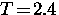K in a magnetic field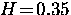T applied parallel to the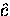-axis. The quality of the fit (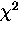) is 4076 for 412 degrees of freedom. The real Fourier transform of both the data and the Gaussian fit are shown in Fig. 4.5(b). The small peak near 47.5 MHz is due to muons which miss the sample and avoid the background suppression system. Typically this constitutes 5 to 15% of the total signal amplitude for our apparatus.

Figure 4.6(a) shows the temperature dependence offor a mosaic of three high quality YBa2Cu3O6.95 single crystals. Despite the poor quality of the fits, the Gaussian function appears to effectively model the change in the second moment of theSR line shape. As the temperature is increased,decreases dramatically due to the natural progression towards a symmetric internal field distribution as the penetration depth grows. A serious drawback with this method is its inability to resolve the several phenomena which separately contribute to the width of theSR line shape. For example, one cannot assume that the linear T dependence of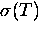at low temperatures necessarily implies that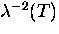has the same linear dependence. For instance, some of the change in the line width may arise from thermal fluctuations of the vortex lines which result in a narrowing of theSR line shape at higher temperatures. Thus, the magnitude of the linear term incould be different than that of, or worse,could have a different leading term.

Consider Fig. 4.6(b), which shows the temperature dependence of the muon depolarization ratein single crystal YBa2Cu3O6.95 for two different applied magnetic fields. There is a distinct drop inat low T when the applied magnetic field is changed from 0.5 to 1.5 T. Moreover, the term linear in T decreases at the higher field. However, there is no way to determine whether the field dependence of the muon depolarization rate is due to intrinsic or extrinsic effects. We now believe that the field dependence ofobserved in some of the earlierSR experiments was misinterpreted as being due to an increase in flux-lattice disorder at low magnetic fields. The precise cause of this field dependence will be addressed later on in this report.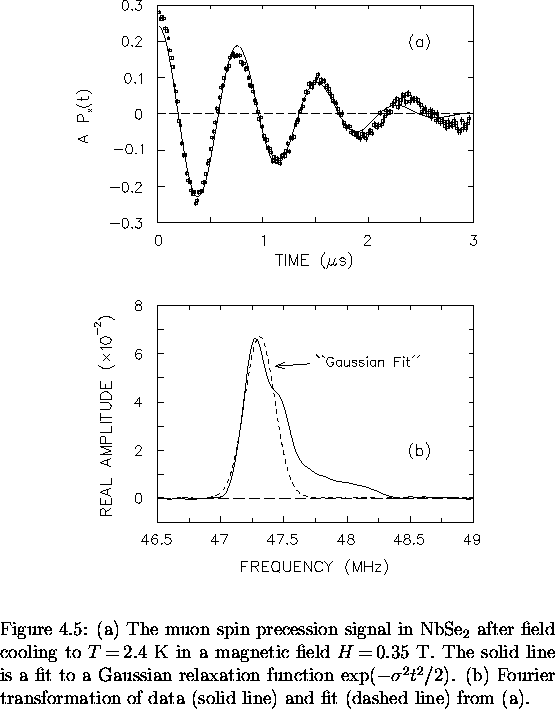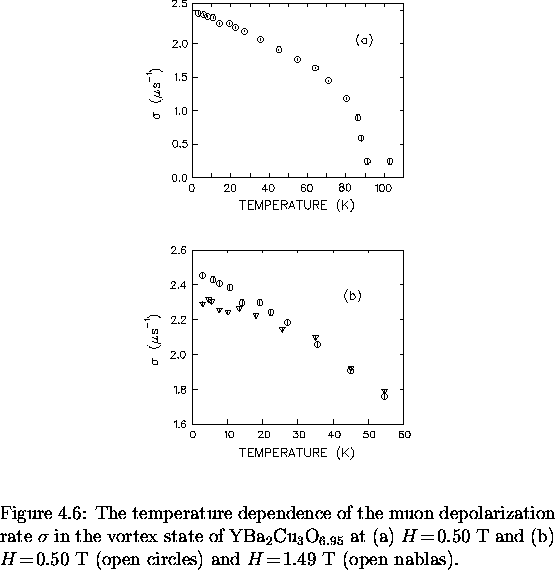There are many other serious limitations or problems associated with using a simple Gaussian analysis. For instance, the shape of the internal field distribution will change when there are variations in the vortex-lattice geometry and at a crossover at low fields where the intervortex spacing L equals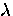. Fitting to a Gaussian function will misidentify these changes in theSR line shape as changes in.This simple analysis is also insensitive to the high-field tail of the measured internal field distribution--so that no information regarding the structure of the vortex cores or the behaviour of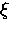can be obtained.Next: 4.4 Field Distribution of Up: 4 Modelling the Internal Previous: 4.2 Pinning, Thermal Fluctuations,